Select a model to view their photos.  To book a photo shoot, click here
L u c a s
V i c t o r i aN e x t  >P h o t o  S h o o t  I n f oAll models were 18 years of age or older during the time of photography. All content and images are in full compliance with the requirements of 18 U.S.C. 2257 and associated regulations.
W e s
P e t e r
D a n i e l
B r e t t
B r a d e n
T i m
C h r i s
J a s o n
S t e p h e n
J o h n
M o r i s s a
D a n i e l  S.
C h a r l i e
M i k e.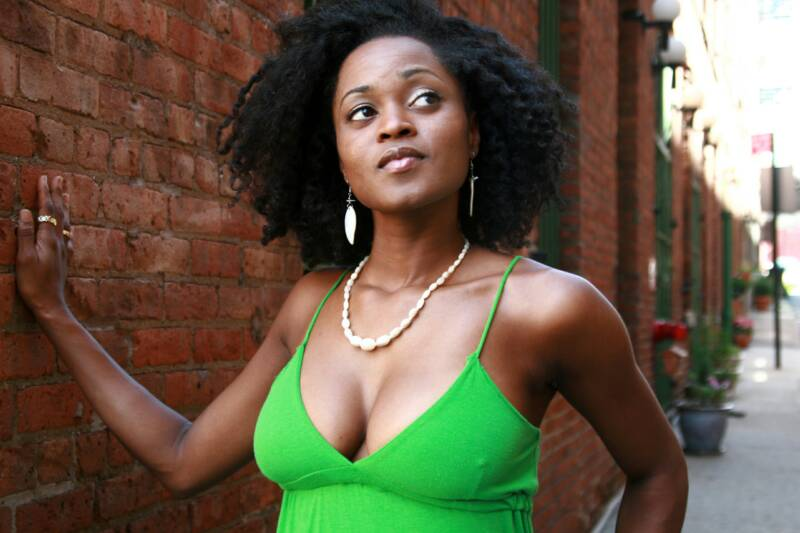G r e t t a
J u s t i nCopyright 1985-2021Nicolas SmithHomeHumanUrbanLandscapesTravelsClientsAbout MeRatesN i c o l a s   S m i t h   P h o t o g r a p h y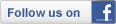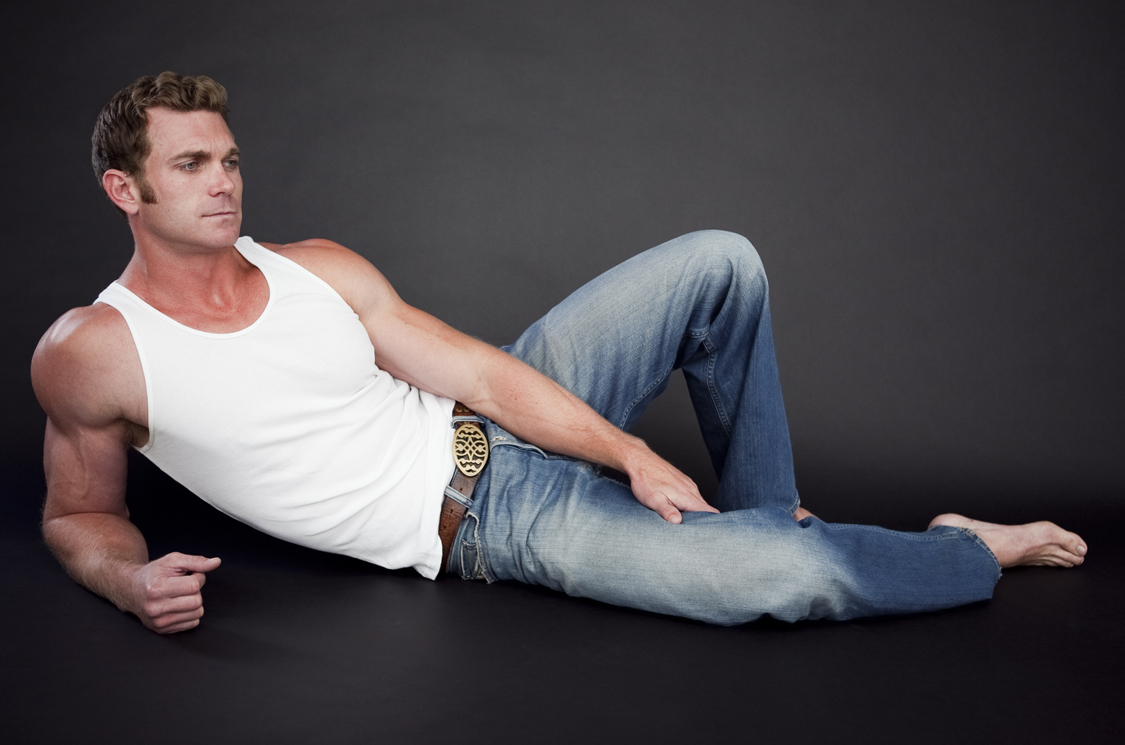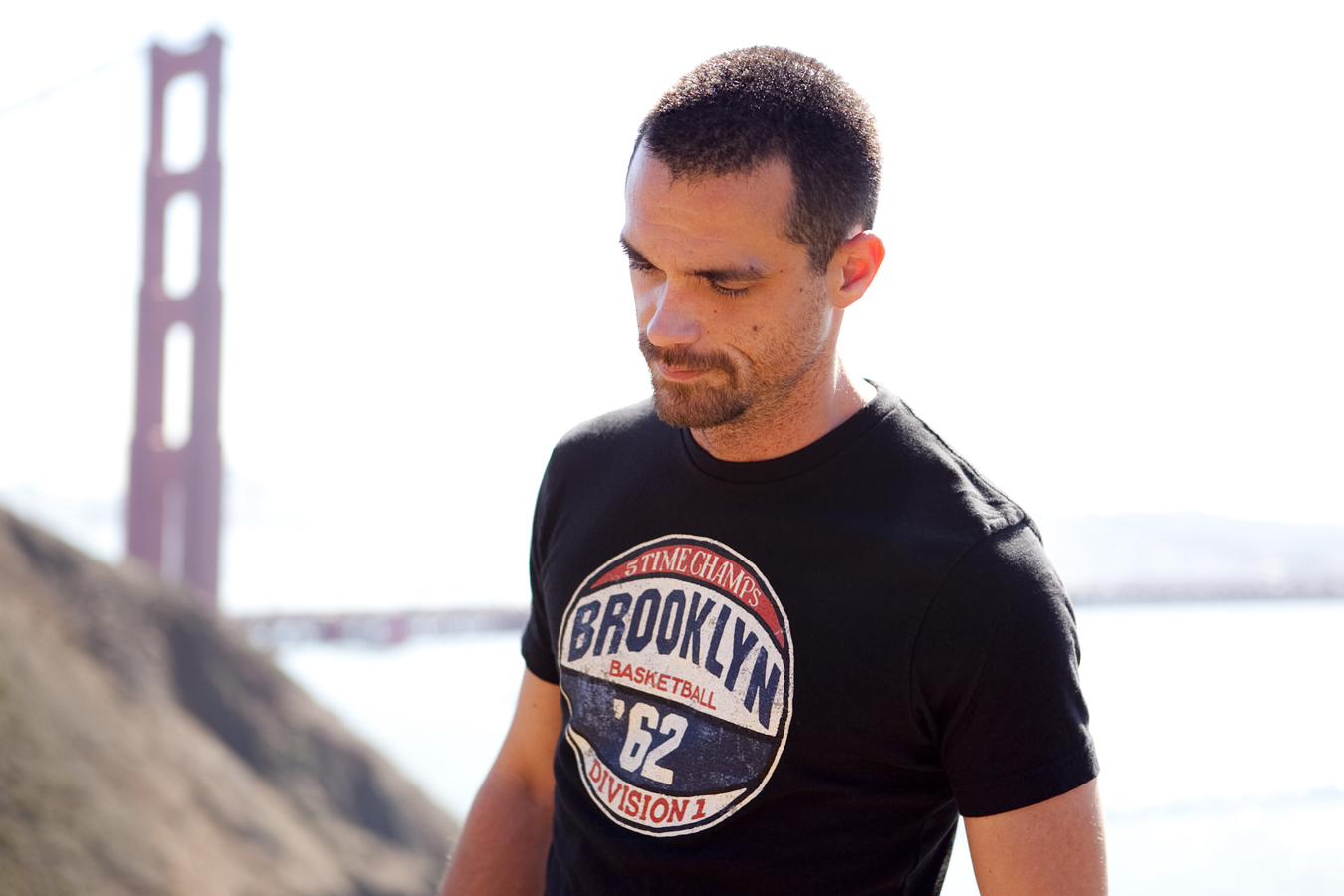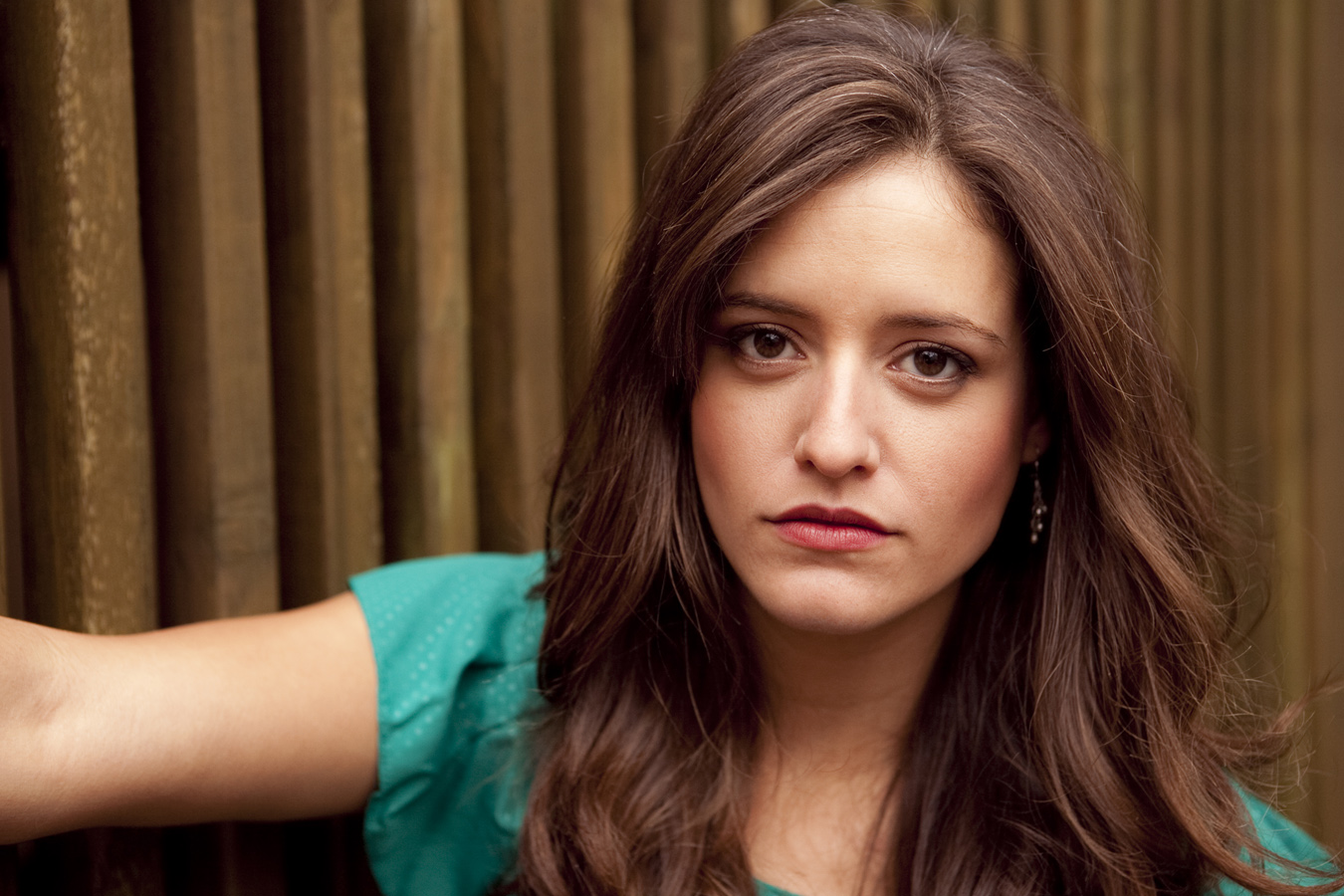N i c o l e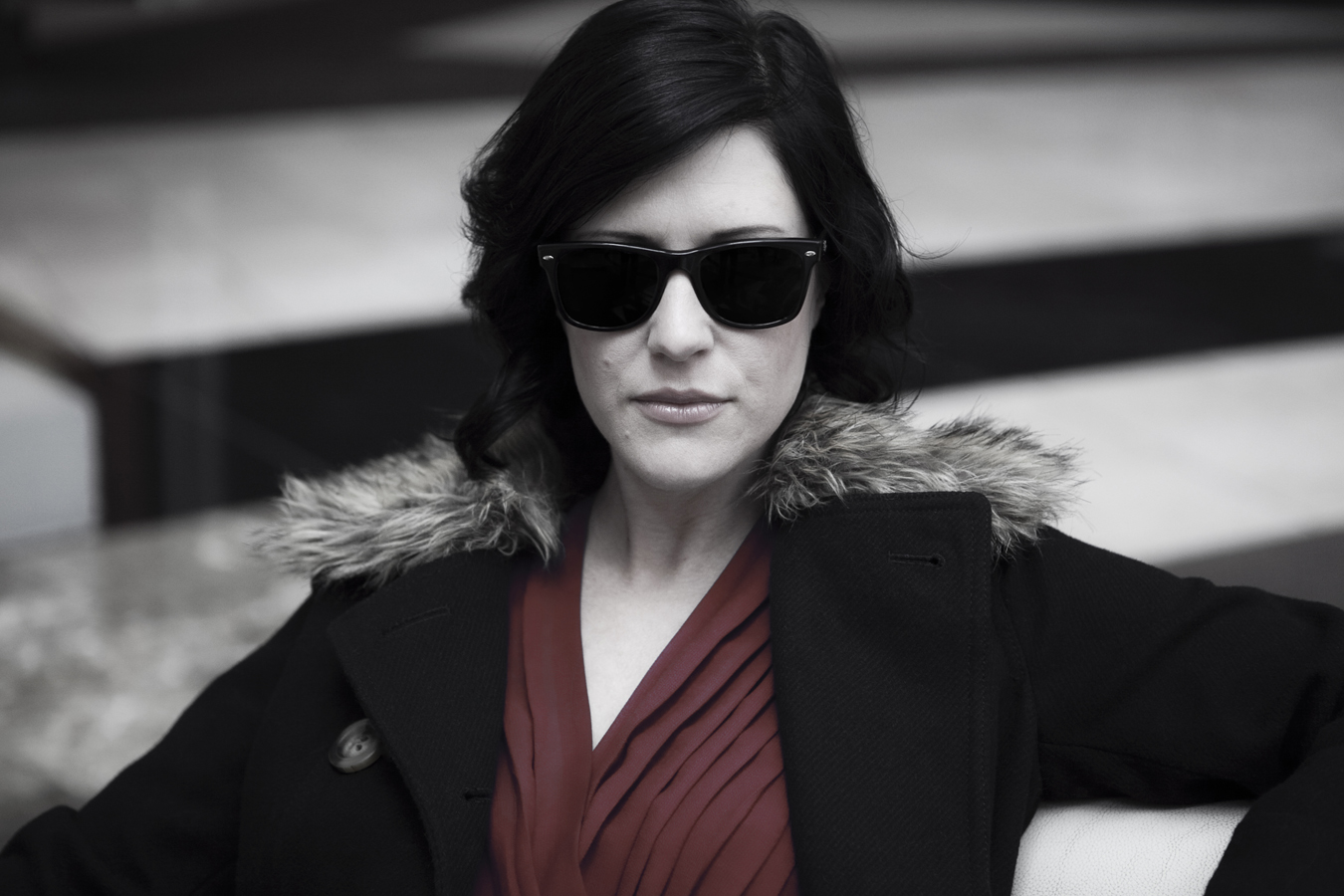K r i s t e n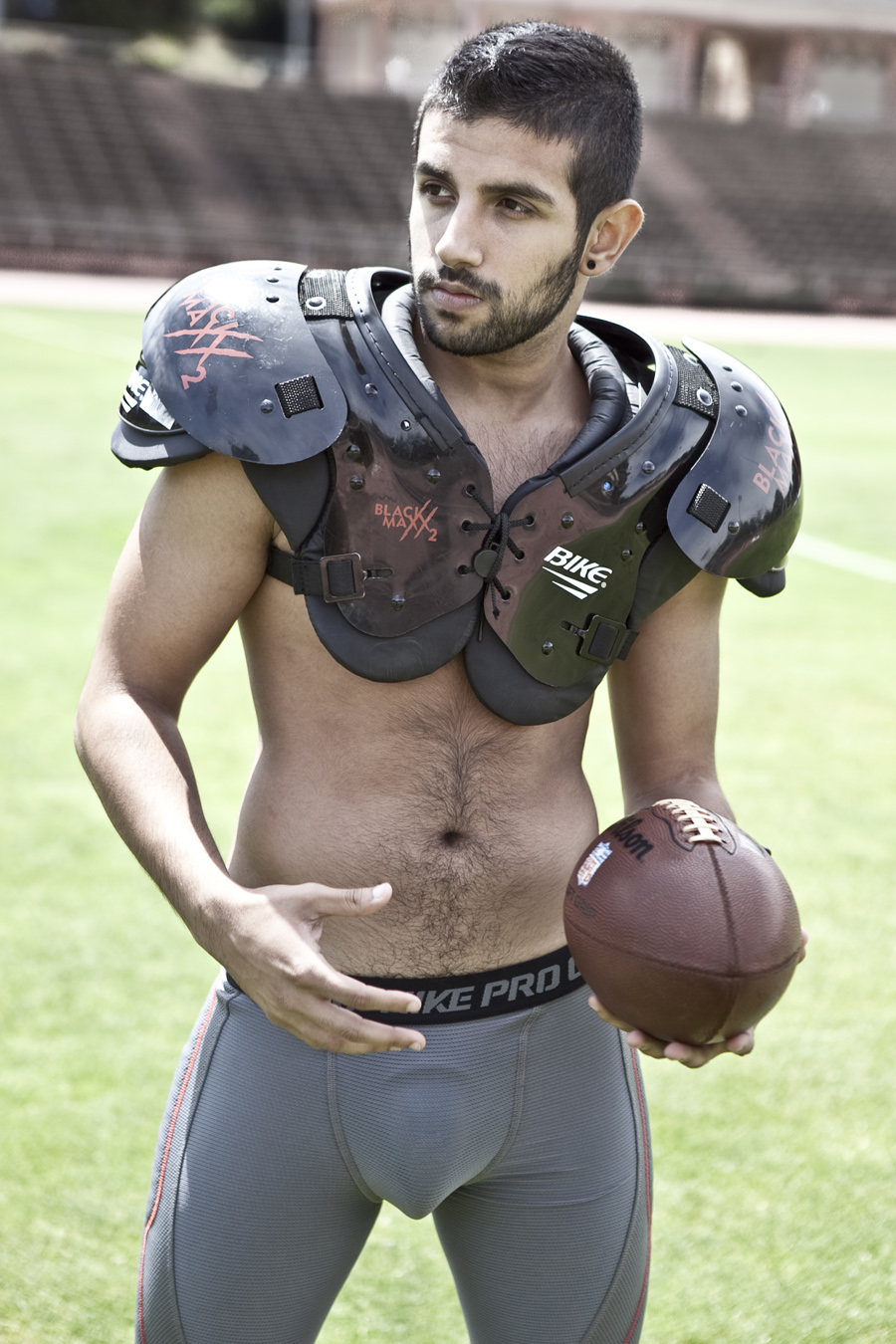M o h a m m a dP a o l o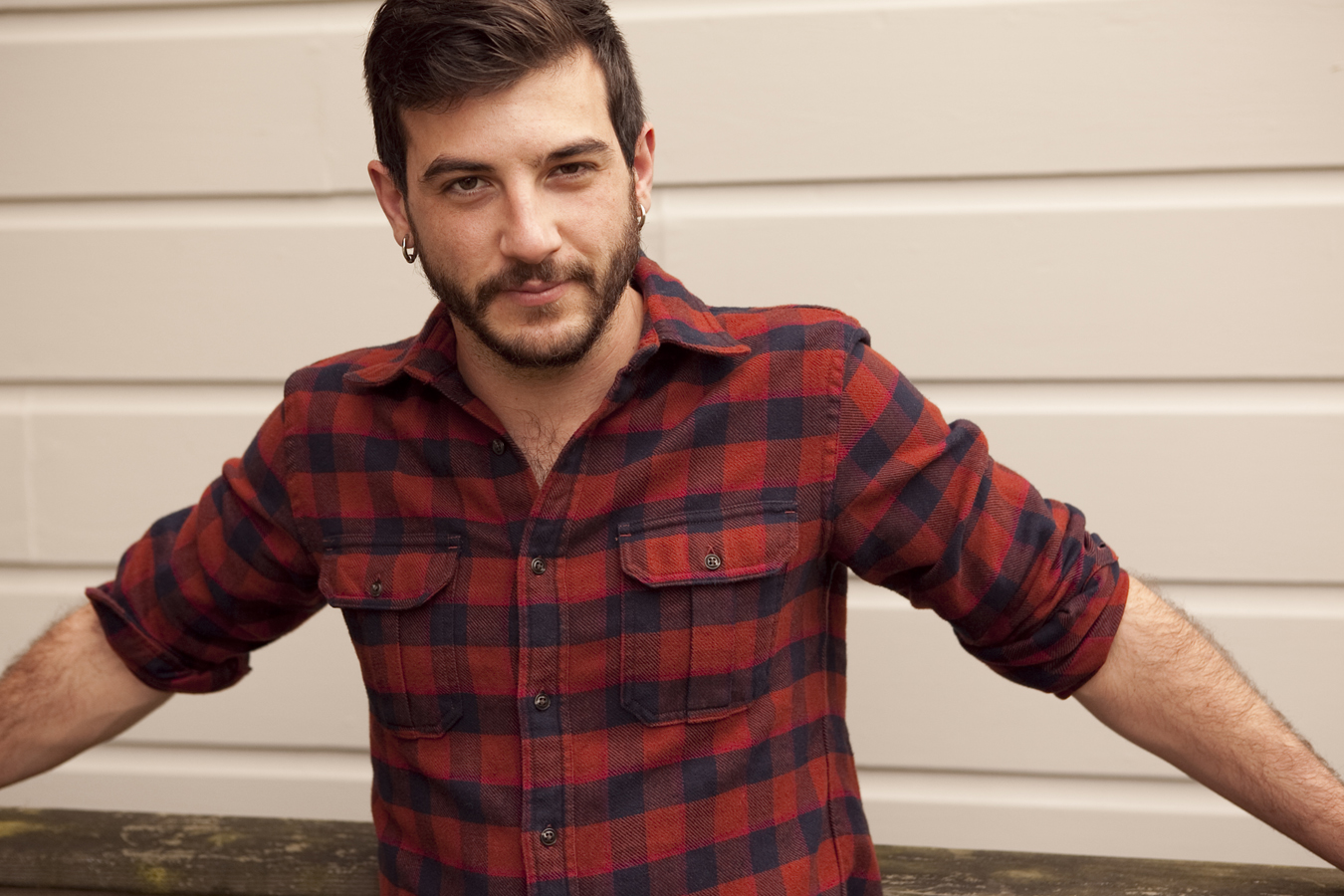R a m s e s